# [转载] $CF725B$ 题解

#include<bits/stdc++.h> using namespace std; long long n,wt; char s; int main() { scanf("%lld",&n); scanf("%c",&s); if(n%4==1) { wt=n*4-4; if(s=='f') wt++; else if(s=='e') wt+=2; else if(s=='d') wt+=3; else if(s=='a') wt+=4; else if(s=='b') wt+=5; else if(s=='c') wt+=6; } else if(n%4==2) { wt=n*4-1; if(s=='f') wt++; else if(s=='e') wt+=2; else if(s=='d') wt+=3; else if(s=='a') wt+=4; else if(s=='b') wt+=5; else if(s=='c') wt+=6; } else if(n%4==3) { wt=n*4-12; if(s=='f') wt++; else if(s=='e') wt+=2; else if(s=='d') wt+=3; else if(s=='a') wt+=4; else if(s=='b') wt+=5; else if(s=='c') wt+=6; } else { wt=n*4-9; if(s=='f') wt++; else if(s=='e') wt+=2; else if(s=='d') wt+=3; else if(s=='a') wt+=4; else if(s=='b') wt+=5; else if(s=='c') wt+=6; } printf("%lld\n",wt); return 0; }

##### 热门推荐
•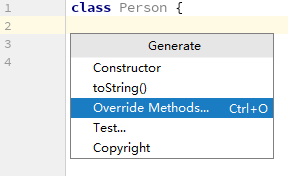浏览(612)
•浏览(390)
•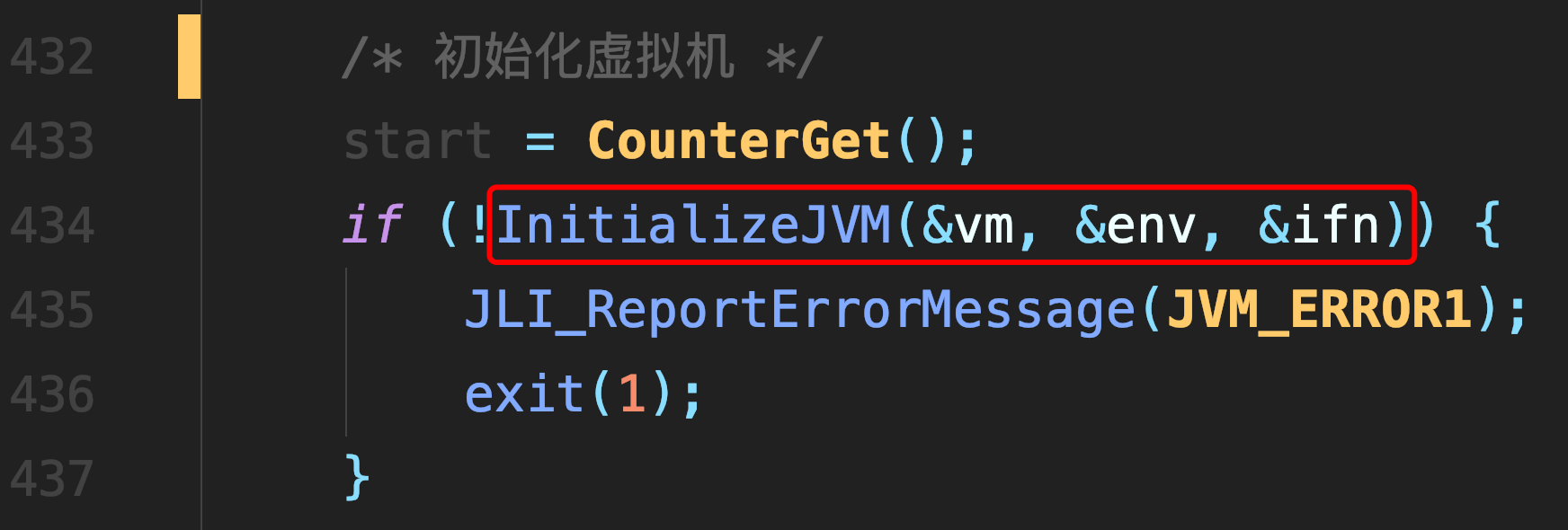浏览(365)
•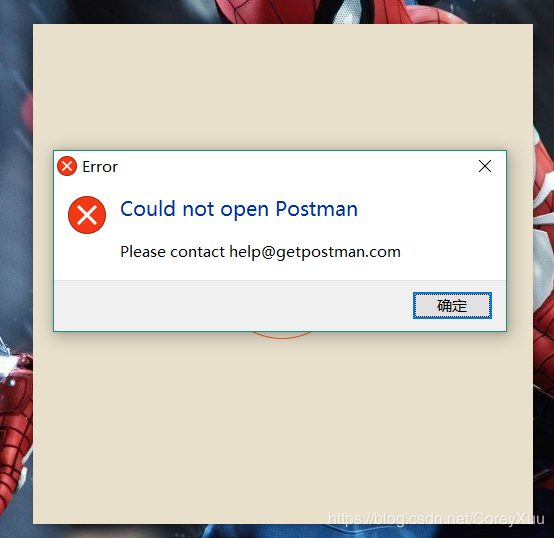浏览(351)
•浏览(347)
•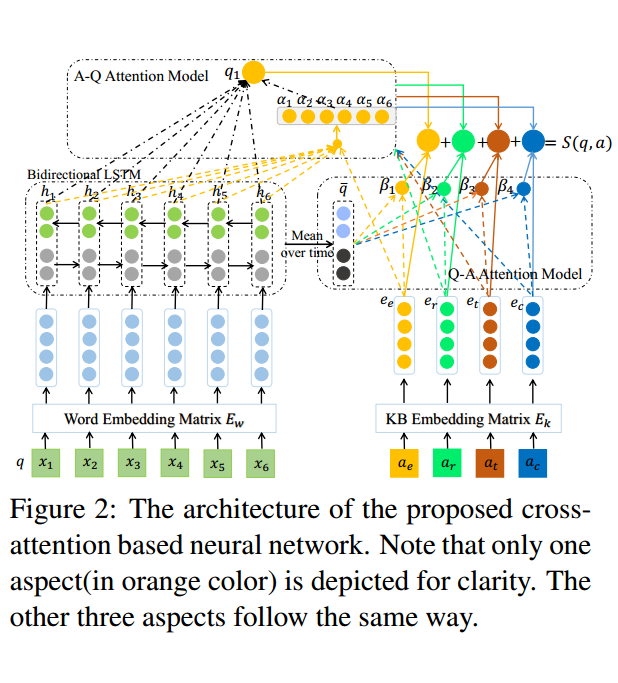浏览(310)
•浏览(292)
•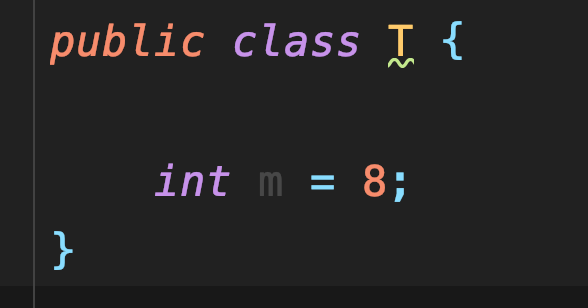浏览(281)
•浏览(267)
•浏览(251)